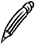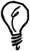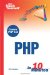# Loops

PHP offers three types of loop constructs that all do the same thingrepeat a section of code a number of timesin slightly different ways.

#### The while Loop

The while keyword takes a condition in parentheses, and the code block that follows is repeated while that condition is true. If the condition is false initially, the code block will not be repeated at all.Infinite Loops The repeating code must perform some action that affects the condition in such a way that the loop condition will eventually no longer be met; otherwise, the loop will repeat forever.

The following example uses a while loop to display the square numbers from 1 to 10:

` \$count = 1; while (\$count <= 10) {   \$square = \$count * \$count;   echo "\$count squared is \$square <br>";   \$count++; } `

The counter variable \$count is initialized with a value of 1. The while loop calculates the square of that number and displays it, then adds one to the value of \$count. The ++ operator adds one to the value of the variable that precedes it.

The loop repeats while the condition \$count <= 10 is true, so the first 10 numbers and their squares are displayed in turn, and then the loop ends.

#### The do Loop

The do loop is very similar to the while loop except that the condition comes after the block of repeating code. Because of this variation, the loop code is always executed at least onceeven if the condition is initially false.

The following do loop is equivalent to the previous example, displaying the numbers from 1 to 10, with their squares:

` \$count = 1; do {   \$square = \$count * \$count;   echo "\$count squared is \$square <br>";   \$count++; } while (\$count <= 10);  `

#### The for Loop

The for loop provides a compact way to create a loop. The following example performs the same loop as the previous two examples:

` for (\$count = 1; \$count <= 10; \$count++) {   \$square = \$count * \$count;   echo "\$count squared is \$square <br>"; } `

As you can see, using for allows you to use much less code to do the same thing as with while and do.

A for statement has three parts, separated by semicolons:

• The first part is an expression that is evaluated once when the loop begins. In the preceding example, you initialized the value of \$count.

• The second part is the condition. While the condition is true, the loop continues repeating. As with a while loop, if the condition is false to start with, the following code block is not executed at all.

• The third part is an expression that is evaluated once at the end of each pass of the loop. In the previous example, \$count is incremented after each line of the output is displayed.

#### Nesting Conditions and Loops

So far you have only seen simple examples of conditions and loops. However, you can nest these constructs within each other to create some quite complex rules to control the flow of a script.Remember to Indent The more complex the flow control in your script is, the more important it becomes to indent your code to make it clear which blocks of code correspond to which constructs.

#### Breaking Out of a Loop

You have already learned about using the keyword break in a switch statement. You can also use break in a loop construct to tell PHP to immediately exit the loop and continue with the rest of the script.

The continue keyword is used to end the current pass of a loop. However, unlike with break, the script jumps back to the top of the same loop and continues execution until the loop condition fails.Sams Teach Yourself PHP in 10 Minutes
ISBN: 0672327627
EAN: 2147483647
Year: 2005
Pages: 151
Authors: Chris Newman

Similar book on Amazon

flylib.com © 2008-2017.
If you may any questions please contact us: flylib@qtcs.net